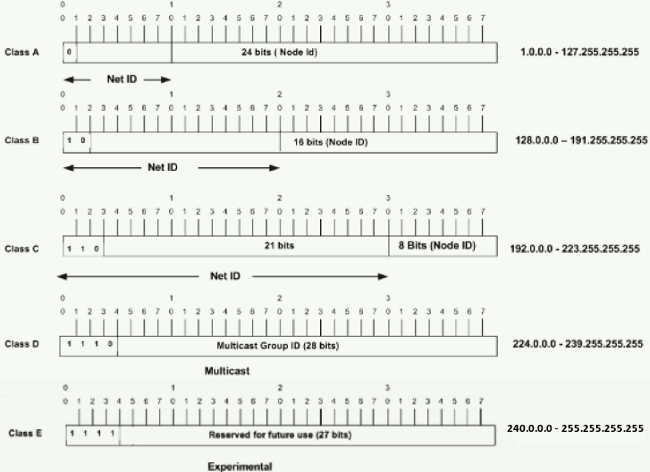# Understanding IP Addresses

An IP address is an address used in order to uniquely identify a device on an IP network. The address is made up of 32 binary bits, which can be divisible into a network portion and host portion with the help of a subnet mask. The 32 binary bits are broken into four octets (1 octet = 8 bits). Each octet is converted to decimal and separated by a period (dot). For this reason, an IP address is said to be expressed in dotted decimal format (for example, 172.16.81.100). The value in each octet ranges from 0 to 255 decimal, or 00000000 – 11111111 binary.

Here is how binary octets convert to decimal: The right most bit, or least significant bit, of an octet holds a value of 20. The bit just to the left of that holds a value of 21. This continues until the left-most bit, or most significant bit, which holds a value of 27. So if all binary bits are a one, the decimal equivalent would be 255 as shown here:

```    1  1  1  1 1 1 1 1
128 64 32 16 8 4 2 1 (128+64+32+16+8+4+2+1=255)```

Here is a sample octet conversion when not all of the bits are set to 1.

```  0  1 0 0 0 0 0 1
0 64 0 0 0 0 0 1 (0+64+0+0+0+0+0+1=65)```

And this is sample shows an IP address represented in both binary and decimal.

```        10.       1.      23.      19 (decimal)
00001010.00000001.00010111.00010011 (binary)```

These octets are broken down to provide an addressing scheme that can accommodate large and small networks. There are five different classes of networks, A to E. This document focuses on addressing classes A to C, since classes D and E are reserved and discussion of them is beyond the scope of this document.

Note: Also note that the terms “Class A, Class B” and so on are used in this document to help facilitate the understanding of IP addressing and subnetting. These terms are rarely used in the industry anymore because of the introduction of classless interdomain routing (CIDR).

Given an IP address, its class can be determined from the three high-order bits. Figure 1 shows the significance in the three high order bits and the range of addresses that fall into each class. For informational purposes, Class D and Class E addresses are also shown.

Figure 1In a Class A address, the first octet is the network portion, so the Class A example in Figure 1 has a major network address of 1.0.0.0 – 127.255.255.255. Octets 2, 3, and 4 (the next 24 bits) are for the network manager to divide into subnets and hosts as he/she sees fit. Class A addresses are used for networks that have more than 65,536 hosts (actually, up to 16777214 hosts!).

In a Class B address, the first two octets are the network portion, so the Class B example in Figure 1 has a major network address of 128.0.0.0 – 191.255.255.255. Octets 3 and 4 (16 bits) are for local subnets and hosts. Class B addresses are used for networks that have between 256 and 65534 hosts.

In a Class C address, the first three octets are the network portion. The Class C example in Figure 1 has a major network address of 192.0.0.0 – 223.255.255.255. Octet 4 (8 bits) is for local subnets and hosts – perfect for networks with less than 254 hosts.

Once you have the address and the mask represented in binary, then identifying the network and host ID is easier. Any address bits which have corresponding mask bits set to 1 represent the network ID. Any address bits that have corresponding mask bits set to 0 represent the node ID.

```8.20.15.1 = 00001000.00010100.00001111.00000001
255.0.0.0 = 11111111.00000000.00000000.00000000
-----------------------------------
net id |      host id

netid =  00001000 = 8
hostid = 00010100.00001111.00000001 = 20.15.1```